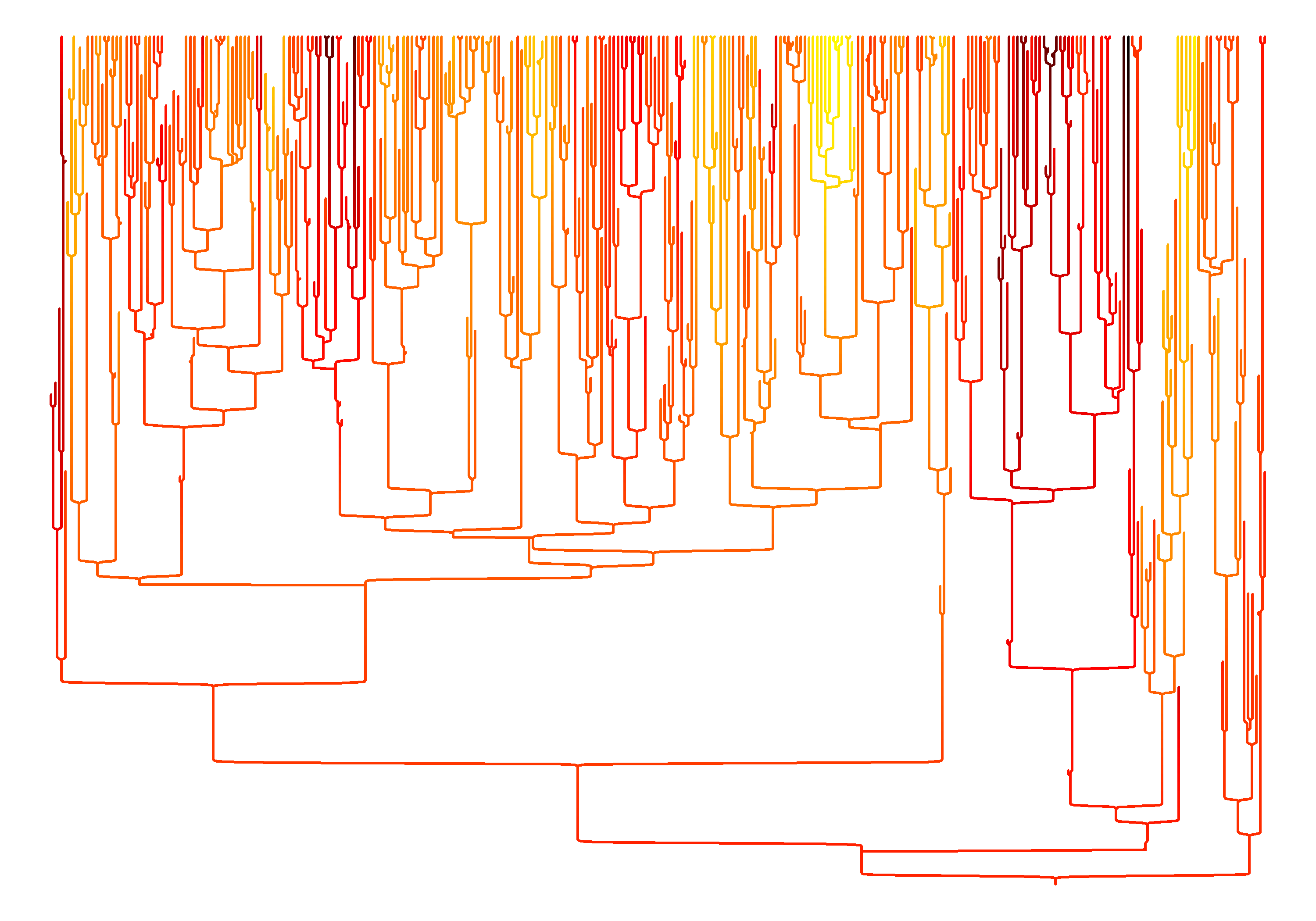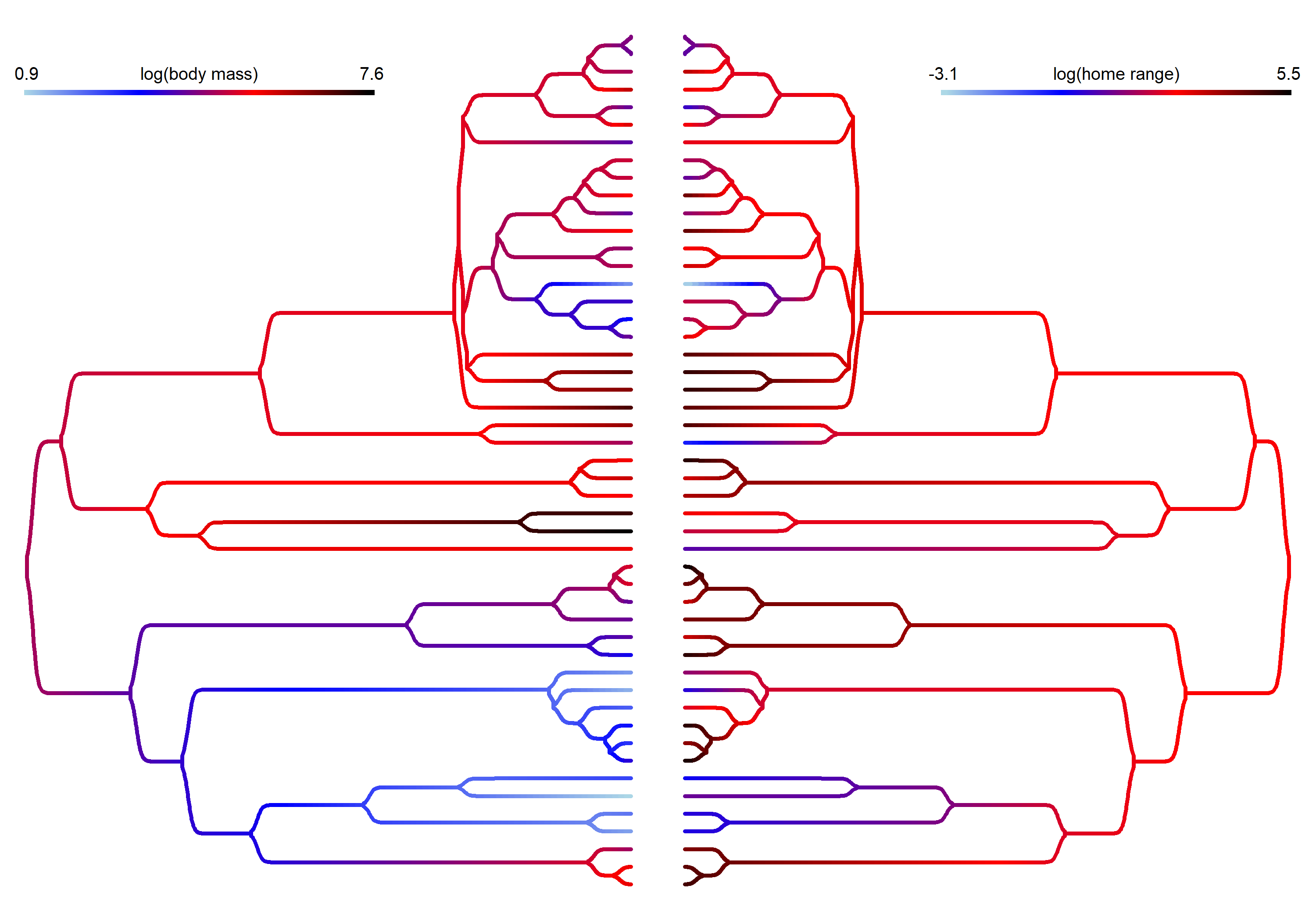## Tuesday, August 30, 2022

### Left/up/down facing trees and tidy tip layout in phytools`sigmoidPhylogram`

Blog readers might have seen my tweet from earlier today indicating that I'd updated the design of this blog.

In the new background for the blog I used an upward facing `sigmoidPhylogram` style tree, with (in the current version) a “tidy” tip layout.

Both of these things are now quite easy to do using the development version of phytools. (Code for the updates is here.)

Let's see.

In the following example I'll simulate a tree with extinction (tidy tip layout only makes sense for non-ultrametric trees), simulate a trait on that tree, create a `"contMap"` object, and then graph it in an “upwards” direction.

``````## load package
library(phytools)
packageVersion("phytools")
``````
``````##  '1.1.15'
``````
``````## simulate a birth-death tree with at least 120
## extant tips
N<-0
while(N<120){
tree<-pbtree(b=1.5,d=0.8,t=4)
N<-length(getExtant(tree))
}
## simulate trait
x<-fastBM(tree)
## create "contMap" object
cMap<-contMap(tree,x,plot=FALSE)
cMap<-setMap(cMap,c("yellow","orange","red","black"))
## plot
plot(tree,type="tidy",show.tip.label=FALSE,plot=FALSE,direction="upwards")
tips<-get("last_plot.phylo",envir=.PlotPhyloEnv)\$xx[1:Ntip(tree)]
sigmoidPhylogram(cMap\$tree,colors=cMap\$cols,ftype="off",lwd=2,tips=tips,
``````Now let's try two right & left facing trees.

In this case I'll use mammal body size & home range size data from Garland et al. (1992).

``````data(mammal.tree)
data(mammal.data)
lnBodyMass<-setNames(log(mammal.data\$bodyMass),rownames(mammal.data))
lnHomeRange<-setNames(log(mammal.data\$homeRange),rownames(mammal.data))
cMap.bodyMass<-contMap(mammal.tree,lnBodyMass,plot=FALSE)
cMap.bodyMass<-setMap(cMap.bodyMass,c("lightblue","blue","red","black"))
cMap.homeRange<-contMap(mammal.tree,lnHomeRange,plot=FALSE)
cMap.homeRange<-setMap(cMap.homeRange,c("lightblue","blue","red","black"))
par(mfrow=c(1,2))
sigmoidPhylogram(cMap.bodyMass\$tree,colors=cMap.bodyMass\$cols,
ftype="off",lwd=3)
lims=cMap.bodyMass\$lims,prompt=FALSE,outline=FALSE,
x=0,y=0.9*par()\$usr,subtitle="",fsize=0.8)
sigmoidPhylogram(cMap.homeRange\$tree,colors=cMap.homeRange\$cols,
ftype="off",lwd=3,direction="leftwards")
title="log(home range)",lims=cMap.homeRange\$lims[2:1],
prompt=FALSE,outline=FALSE,x=0,y=0.9*par()\$usr,subtitle="",
fsize=0.8)
``````One thing you might have noticed is that I had to flip the direction of my color legend for home range size. This is because in `sigmoidPhylogram` rightward facing trees are just plotted leftward with the x-axis flipped. (Ditto for upwards vs. downwards.)

That's it.